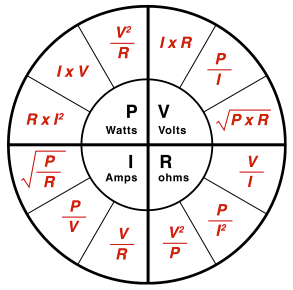# Ohm’s Law Calculator

Use this tool to calculate relationships between current, voltage, resistance, and power in resistive circuits.

Enter two input values and click calculate to solve for the remaining values. Reset after each calculation for best results.

+More

Voltage (V)
V
Current (I)
A
Resistance (R)
Ω
Power (P)
W×